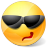# Math Is Fun Forum

Discussion about math, puzzles, games and fun.   Useful symbols: ÷ × ½ √ ∞ ≠ ≤ ≥ ≈ ⇒ ± ∈ Δ θ ∴ ∑ ∫  π  -¹ ² ³ °

You are not logged in.

## #1 2012-07-17 13:28:06

careless25
Real MemberRegistered: 2008-07-24
Posts: 560

### Local min/max of multivariable function

A circular hot plate given by the relationship x^2 + y^2 <= 4 is heated according to the spatial temperature function T(x, y) = 10-x^2 + 2x - 4y^2. Find the hottest and coldest temperatures on the plate and the points at which they occur.

Here is what I have so far:
g(x,y) = x^2 + y^2 - K where K<= 4

del f = (-2x + 2, -8y)
del g = (2x, 2y)

Applying LaGrange multiplier

1) -2x+2 = lambda(2x)
2) -8y = lambda(2y)
3) x^2 + y^2 = K

solving for eqn 2)
we get y=0 or lamba = -4

sub lambda = -4 in eqn 1
we get x = -1/3

now I am stuck, since there are infinite values of K we have to solve for. I know there is going to be points (-1/3, +/- y) and (+/-x, 0).

Another approach that gives me half the answer is solving for the global max of T(x,y), which coincidentally lies in the constraint given, but then I am at a loss at finding the local min.

Any ideas?

Offline

## #2 2012-07-17 19:39:23

BobRegistered: 2010-06-20
Posts: 9,278

### Re: Local min/max of multivariable function

hi careless25

I get Tmax = 11 at (1,0)

There isn't a global min within the range, in fact there won't be one for any limited range of x and y because T decreases as x and y get larger, indefinitely.

So I argued like this:

(i) Pick the value of y that makes T as small as possible.  y=2

(ii) Use that for T  .  T = -x^2 + 2x -6

(iii) Find the lowest T.  As this quadratic gets smaller as x gets larger that will be when x = 0.  (0,2)  gives Tmin  = -6

I've checked this out in Excel using a range of values and confirmed both answers numerically.

LATER EDIT AFTER READING POST #4

I seem to have forgotten negative values so this is NOT the minimum.

Bob

ps.  Thinking about it some more, I think this only works for these functions of x and y.  You could probably construct a quadratic in x that doesn't have it's minimum when y has its minimum  (by making the x^2 term positive for instance) so this approach wouldn't always work.

Children are not defined by school ...........The Fonz
You cannot teach a man anything;  you can only help him find it within himself..........Galileo Galilei
Sometimes I deliberately make mistakes, just to test you!  …………….BobOffline

## #3 2012-07-17 20:18:43

bobbym
bumpkinFrom: Bumpkinland
Registered: 2009-04-12
Posts: 109,606

### Re: Local min/max of multivariable function

Hi careless25;

I have never seen a Lagrangian Multiplier used on an inequality. Usually the constraints and the function to be minimized/maximized are equations.

1) -2x+2 = lambda(2x)
2) -8y = lambda(2y)
3) x^2 + y^2 = K

Mathematica can take us to a complete solution to this but I do not understand it.

In mathematics, you don't understand things. You just get used to them.
If it ain't broke, fix it until it is.
Always satisfy the Prime Directive of getting the right answer above all else.

Offline

## #4 2012-07-18 00:54:07

TheDude
Member
Registered: 2007-10-23
Posts: 361

### Re: Local min/max of multivariable function

I think this is right.

First take the partial derivatives of T(x, y) and set them equal to 0:

Which agrees with Bob's answer.  This is the only critical point for T(x, y) in the region x^2 + y^2 <= 4 (indeed, it's the only critical point anywhere).  This means that to find the minimum of T(x, y) in this region we only need to consider the boundary x^2 + y^2 = 4.

Find the critical point:

Now we have just 3 points to check by hand, the boundary cases (-2, 0) and (2, 0) and the critical point (-1/3, +- sqrt{35/9}):

So the minimum temperature is -57/9 and occurs at (-1/3, +- sqrt{35/9})

Wrap it in bacon

Offline

## #5 2012-07-18 01:14:17

BobRegistered: 2010-06-20
Posts: 9,278

### Re: Local min/max of multivariable function

Thanks The Dude,

For some reason I completely forgot about negative values of x.  Your answer looks much better to me.Bob

Children are not defined by school ...........The Fonz
You cannot teach a man anything;  you can only help him find it within himself..........Galileo Galilei
Sometimes I deliberately make mistakes, just to test you!  …………….BobOffline

## #6 2012-07-18 02:47:57

careless25
Real MemberRegistered: 2008-07-24
Posts: 560

### Re: Local min/max of multivariable function

TheDude,

How do we know that we just have to consider the boundary points? What if there was a graph whos min was not on the  boundary point?

Thanks

Offline

## #7 2012-07-18 03:14:36

TheDude
Member
Registered: 2007-10-23
Posts: 361

### Re: Local min/max of multivariable function

If it wasn't on a boundary point then it would be at a critical point, and we already know that there is only 1 critical point for T(x, y) and that it is a global maximum.

Wrap it in bacon

Offline